×

Search anything:

# SMOTE for Imbalanced Dataset

#### Machine Learning (ML)Get this book -> Problems on Array: For Interviews and Competitive Programming

Reading time: 30 minutes | Coding time: 10 minutes

In this post, we will see how to deal with an imbalanced dataset using SMOTE (Synthetic Minority Over-sampling TEchnique). We will also see its implementation in Python.

## Imbalanced Dataset

An individual in the domain of Machine Learning is likely to come across a dataset where the class labels distribution is significantly different. In simple words, Imbalanced Dataset usually reflects an unequal distribution of classes within a dataset.

The term accuracy can be highly misleading as a performance metric for such data.

Consider a dataset with 1000 data points having 950 points of class 1 and 50 points of class 0. If we have a model which predicts all observations as 1, the accuracy in such case would be 950/1000= 95%.

However, is it really a good model? A BIG NO :)

In such cases, we should analyze the Recall, Precision and F1-scores depending on the business requirements. Consider an example of cancer detection; here, False Negatives are of primary concern. The value of false negatives should be as low as possible since we do not want our model to predict a cancerous patient as non-cancerous.

A similar scenario happens in the case of detecting whether a given transaction is fraud or not. These metrics can be calculated using the following forumulas.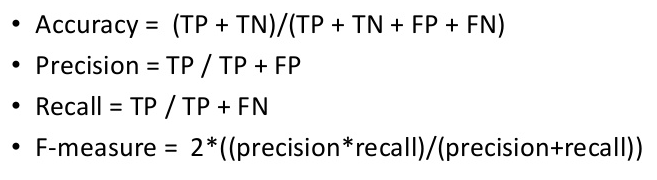## Dealing with Imbalanced Dataset

There exists a bunch of sampling techniques to deal with imbalanced data, which are primarily classified into-

### Under-sampling

• In this sampling technique, the samples of the majority class are randomly removed to match the proportion of distribution when compared to the minority class.
• It is generally not preferred since we are losing valuable information just to match the proportion of 2 classes and may lead to bias.

### Over-sampling

• In this technique, we increase the samples of minority class to make samples of both minor and major classes as equal.
• One possible way is to replicate the samples of the minority class, and the other possible method is to generate some synthetic points using SMOTE.
• The random over-sampling(replicating minority samples) is not preferred since it can lead to overfitting due to copying the same information.

## What is SMOTE?

• SMOTE stands for Synthetic Minority Over-sampling TEchnique.
• It is an over-sampling technique in which new synthetic observations are created using the existing samples of the minority class.
• It generates virtual training records by linear interpolation for the minority class.
• These synthetic training records are generated by randomly selecting one or more of the k-nearest neighbours for each example in the minority class.
• After the oversampling process, the data is reconstructed, and several classification models can be applied for the processed data.

The various steps involved in SMOTE are-

Step 1: Setting the minority class set A, for each $x \in A$, the k-nearest neighbors of x are obtained by calculating the Euclidean distance between x and every other sample in set A.

Step 2: The sampling rate N is set according to the imbalanced proportion. For each $x \in A$, N examples (i.e x1, x2, …xn) are randomly selected from its k-nearest neighbors, and they construct the set $A_1$ .

Step 3: For each example $x_k \in A_1$ (k=1, 2, 3…N), the following formula is used to generate a new example:
$x' = x + rand(0, 1) * \mid x - x_k \mid$
where rand(0, 1) denotes a random number between 0 and 1.

• First, the initial distribution of the minority class is shown.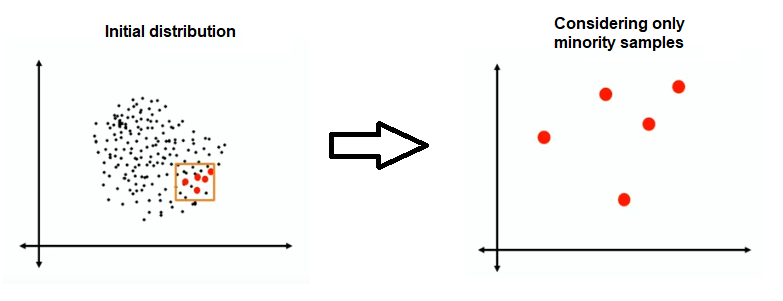• Say if the value of k nearest neighbour is 2. Each point will find its 2 nearest neighbours (say using euclidean distance). If we consider only point A initially, then B and C are its nearest neighbours.

• By using step 3 in the above algorithm, new synthetic points are generated. It is not necessary to generate a single synthetic point on each line; it rather depends on the number of synthetic samples required. A single line can accommodate multiple synthetic points as well.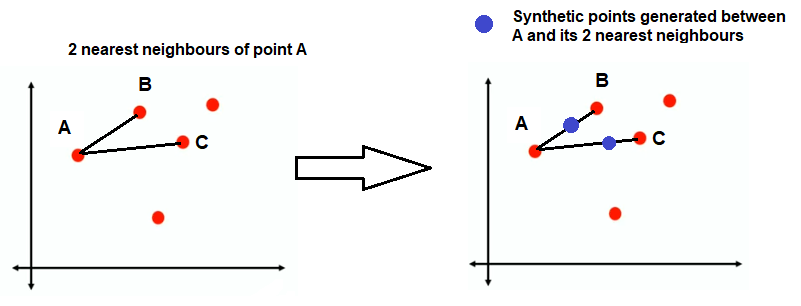• Similarly, all points are considered, and synthetic observations are generated in a similar fashion for them also.

## Implementation in Python

It is very easy to incorporate SMOTE using Python. We only have to install the imbalanced-learn package.

pip install imblearn


The dataset used is of Credit Card Fraud Detection from Kaggle and can be downloaded from here.

• Importing necessary packages
import numpy as np
import pandas as pd

from sklearn.model_selection import train_test_split
from sklearn.linear_model import LogisticRegression
from sklearn.metrics import accuracy_score,classification_report,confusion_matrix
import seaborn as sns

df = pd.read_csv('creditcard.csv')

• Analyzing class distribution
class_dist=df['Class'].value_counts()
print(class_dist)
print('\nClass 0: {:0.2f}%'.format(100 *loan_status_dist / (class_dist+class_dist)))
print('Class 1: {:0.2f}%'.format(100 *loan_status_dist / (class_dist+class_dist)))


Class 0: 99.83%

Class 1: 0.17%

• Splitting data into train and test test
X = df.drop(columns=['Time','Class'])
y = df['Class']

x_train,x_test,y_train,y_test = train_test_split(X,y,random_state=100,test_size=0.3,stratify=y)

• Evaluating results without SMOTE
model = LogisticRegression()
model.fit(x_train,y_train)
pred = model.predict(x_test)
print('Accuracy ',accuracy_score(y_test,pred))
print(classification_report(y_test,pred))
sns.heatmap(confusion_matrix(y_test,pred),annot=True,fmt='.2g')


If the recall measure is of main concern, we see that its value is only 0.59 and it certainly needs to be improved.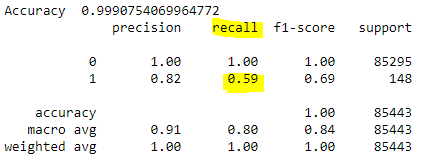• Here we use the SMOTE module from imblearn

k_neighbours- represents number of nearest to be consider while generating synthetic points.

sampling_strategy- by default generates synthetic points equal to number of points in majority class. Since, here it is 0.5 it will generate synthetic points half of that of majority class points.

random_state- is simply for reproducability purpose.

print("Before OverSampling, counts of label '1': {}".format(sum(y_train == 1)))
print("Before OverSampling, counts of label '0': {} \n".format(sum(y_train == 0)))

from imblearn.over_sampling import SMOTE

sm = SMOTE(sampling_strategy=0.5,k_neighbors=5,random_state = 100)
X_train_res, y_train_res = sm.fit_sample(x_train, y_train.ravel())

print('After OverSampling, the shape of train_X: {}'.format(X_train_res.shape))
print('After OverSampling, the shape of train_y: {} \n'.format(y_train_res.shape))

print("After OverSampling, counts of label '1': {}".format(sum(y_train_res == 1)))
print("After OverSampling, counts of label '0': {}".format(sum(y_train_res == 0)))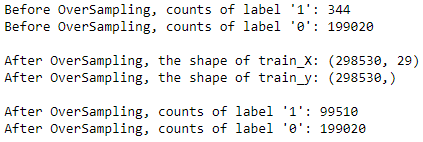• Results of SMOTE
lr = LogisticRegression()
lr.fit(X_train_res, y_train_res.ravel())
predictions = lr.predict(x_test)

print('Accuracy ',accuracy_score(y_test,predictions))
print(classification_report(y_test, predictions))
sns.heatmap(confusion_matrix(y_test,predictions),annot=True,fmt='.2g')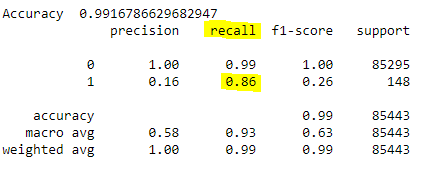We can clearly see, the Recall value has significantly improved by using the SMOTE technique.

## Conclusion

In this post we looked at why accuracy is not a good metric for an imbalanced data sceanario. After that we saw different sampling techniques (Under & Over sampling). We then looked at a deatiled explanation of SMOTE and its implementation in Python.#### Akshat Maheshwari

Machine Learning Developer, Intern at OpenGenus | Integrated B. Tech and M. Tech student at ABV-Indian Institute of Information Technology and Management (2016 to 2021)

SMOTE for Imbalanced Dataset